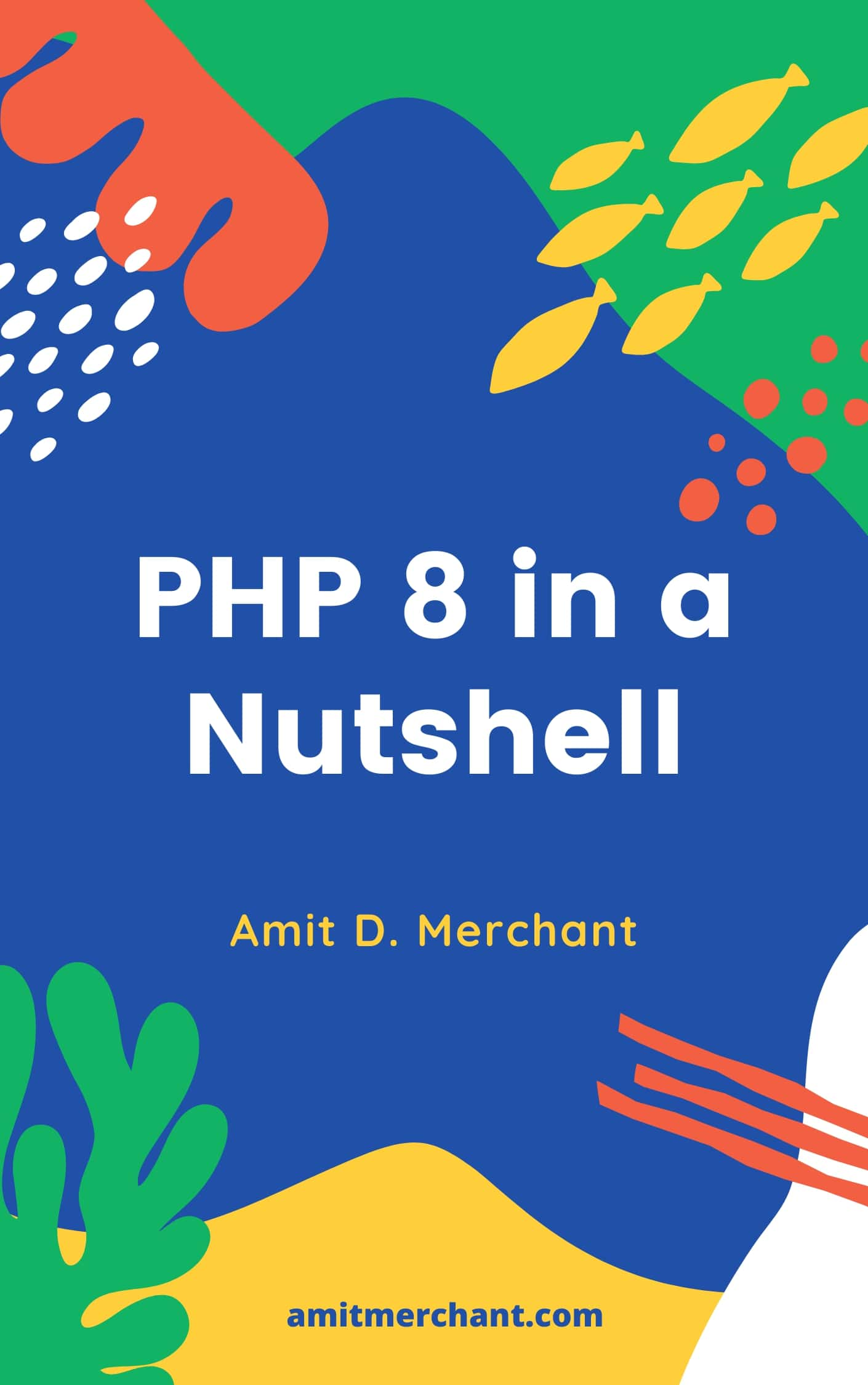# Amit MerchantVerified (\$10/year for the domain)

A blog on PHP, JavaScript, and more

# A simple clamp() function in PHP

If you have worked with CSS, you may have come across this function called clamp() that clamps a value between an upper and lower bound.

In CSS, it can be used to clamp the value of, let’s say, the font’s size based on the viewport’s width to make it dynamic.

Here’s the definition of the function: `clamp(MIN, VAL, MAX)`. Let’s understand this with an example.

``````p {
font-size: clamp(1rem, 2.5vw, 2rem);
}
``````

Essentially, the `clamp()` function will for three things:

• If the `VAL` falls under `MIN` and `MAX`, it will return `VAL`.
• If the `VAL` is greater than `MAX`, it will return `MAX`.
• If the `VAL` is less than `MIN`, it will return `MIN`.

So, in the example above, the `clamp()` function calculates the value of `2.5vw` and adjusts the `font-size` based on the above three conditions.

## Clamp implementation in PHP

I wrote a simple `clamp()` function in PHP which works exactly the same as CSS’s `clamp()` function.

Here’s what the implementation looks like.

``````/**
* clamp checks if an int|float value is within a certain bound.
*
* If the value is in range it returns the value,
* if the value is not in range it returns the nearest bound.
*/
function clamp(int|float \$value, int|float \$min, int|float \$max)
{
if (\$min > \$max) {
throw new Error('min can not be greater than max');
}

if (\$value < \$min) {
return \$min;
} else if (\$value > \$max) {
return \$max;
} else {
return \$value;
}
}

var_dump(clamp(3, 1, 5)); // 3
var_dump(clamp(1, 2, 5)); // 2
var_dump(clamp(6, 1, 5)); // 5
``````

As you can tell, the function is pretty simple. I have changed the order of arguments a little bit but under the hood, the implementation is more or less the same.

A clamp function in PHP can come in handy in scenarios where we want to bind the value of the certain variable in a range.

For instance, in a SaaS application, you could use this function to bind the user’s API usage to a certain range. The example is somewhat broad but you get the idea, right?

👋 Hi there! I'm Amit. I write articles about all things web development. If you like what I write and want me to continue doing the same, I would like you buy me some coffees. I'd highly appreciate that. Cheers!(Includes PHP 8.1 and 8.2)

My Tiny YouTube Channel ➔